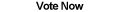Home Articles Components Code Snippets Links# C# Rounding Doubles

## How to Round Doubles

The simplest way to round doubles and decimals in C# is to use the Math.Round function provided by the .NET Framework. Rounding is done to convert a decimal number into the closest whole number (integers in C#).

The most common form of rounding is to round away from zero when an decimal place is 5 or more. However there is more than one way to round that is more effective than others.

## Away From Zero

Rounding away from zero is the first way most people learn how to round. The rule is to round to the next integer away from zero when a decimal is exactly between two integers. Thus 4.5 is rounded up to 5 and -4.5 is rounded down to -5. There are two quick ways to round this way in C#.NET:

```myDouble = (int)(myDouble + 0.5);
myDouble = Math.Round(myDouble, MidpointRounding.AwayFromZero);```

The first line works because casting to an integer automatically drops any decimal places (without any rounding), by adding 0.5, decimal places exactly in the middle are rounded up to the next whole number.

The second line returns the same result. However notice that a MidpointRounding parameter is necessary. That is because .NET Framework uses by default Bankers' Rounding.

## Bankers' Rounding

Rounding to the nearest even number is known as Bankers' Rounding. This method of rounding works exactly as it sounds, when a decimal point is exactly between two whole numbers, it rounds to the nearest whole even number. So 3.5 is rounded to 4, but 4.5 is also rounded to 4.

Bankers' Rounding is the default in C#.NET. So the two lines below give the same result:

```myDouble = Math.Round(myDouble);
myDouble = Math.Round(myDouble, MidpointRounding.ToEven);```

This method of rounding might be strange at first, but it is a better method of rounding in most cases. Bankers' Rounding aims to round up and round down the same number of times in a large set of numbers. Unlike rounding away from zero (which always rounds up), Bankers' Rounding generates more accurate results.

## Rounding C# Example

Let's take a set of 10 random doubles (with only one decimal place): 2.9, 5.8, 9.7, 1.3, 8.8, 8.4, 2.1, 2.3, 3.3, 4.7

Now we can calculate three values: the actual sum, the sum of each number rounded away from zero, and the sum of each rounded to the nearest even number (Bankers' Rounding).

The actual sum comes out to 49.3
The sum rounding away from zero is 49
The sum rounding to the nearest even number is 49

The results for both rounding methods are the same and are close to the actual result. Why? Take a look at the numbers, all the doubles are fairly close to an integer. Thus we don't really have to worry about a bias in this case.

Now let's modify our random sample to make each double be exactly between to integers: 2.5, 5.5, 9.5, 1.5, 8.5, 8.5, 2.5, 2.5, 3.5, 4.5

In this case the actual sum comes out to 49
The sum rounding away from zero is 54
The sum rounding to the nearest even number is 48

Now which number is closest to the actual result? Not only is the first rounding method off, it is way off. This is because by constantly rounding up, we create a bias in the final sum.

In this case the math operation performed is a simple sum. Once you start using more advanced mathematics and statistics, a bias can yield extremely inaccurate results. This is why Bakers' Rounding is the default in C#.NET.

In conclusion, rounding in .NET is simple. But knowning a few details about the data and the intention of rounding can allow C# developers to choose the more suited method of rounding. In most cases, rounding to the nearest even whole number is the best way to go.

Back to C# Article List